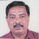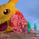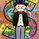90302 views
LazyBear's wavetrend oscillator enhanced with wavetrend cross visualization on the indicator as well as with bar color highlights.
Open-source script

In true TradingView spirit, the author of this script has published it open-source, so traders can understand and verify it. Cheers to the author! You may use it for free, but reuse of this code in a publication is governed by House Rules. You can favorite it to use it on a chart.

Want to use this script on a chart?
```//
// @author LazyBear
//
// If you use this code in its original/modified form, do drop me a note.
//
study(title="WaveTrend with Crosses [LazyBear]", shorttitle="WT_CROSS_LB")
n1 = input(10, "Channel Length")
n2 = input(21, "Average Length")
obLevel1 = input(60, "Over Bought Level 1")
obLevel2 = input(53, "Over Bought Level 2")
osLevel1 = input(-60, "Over Sold Level 1")
osLevel2 = input(-53, "Over Sold Level 2")

ap = hlc3
esa = ema(ap, n1)
d = ema(abs(ap - esa), n1)
ci = (ap - esa) / (0.015 * d)
tci = ema(ci, n2)

wt1 = tci
wt2 = sma(wt1,4)

plot(0, color=gray)
plot(obLevel1, color=red)
plot(osLevel1, color=green)
plot(obLevel2, color=red)
plot(osLevel2, color=green)

plot(wt1, color=green)
plot(wt2, color=red)
plot(wt1-wt2, color=blue, style=area, transp=80)
plot(cross(wt1, wt2) ? wt2 : na, color = black , style = circles, linewidth = 3)
plot(cross(wt1, wt2) ? wt2 : na, color = (wt2 - wt1 > 0 ? red : lime) , style = circles, linewidth = 2)
barcolor(cross(wt1, wt2) ? (wt2 - wt1 > 0 ? aqua : yellow) : na)
```jfeste512
@jfeste512, according to my understanding they are probable buy and sell indicators (respectively) but with confirmation of following next candle.Couple small changes. Removed the default colouring and titled each section for easier alerts. Also added an option to set an alert when the wavetrend is reversing.

n1 = input(10, "Channel Length")
n2 = input(21, "Average Length")
obLevel1 = input(60, "Over Bought Level 1")
obLevel2 = input(53, "Over Bought Level 2")
osLevel1 = input(-60, "Over Sold Level 1")
osLevel2 = input(-53, "Over Sold Level 2")

ap = hlc3
esa = ema(ap, n1)
d = ema(abs(ap - esa), n1)
ci = (ap - esa) / (0.015 * d)
tci = ema(ci, n2)

wt1 = tci
wt2 = sma(wt1,4)

plot(0, title="Zero Line")
plot(obLevel1, title="Overbought Lv.1")
plot(obLevel2, title="Overbought Lv.2")
plot(osLevel1, title="Oversold Lv.1")
plot(osLevel2, title="Oversold Lv.2")

plot(wt1, title="WT Bull Line")
plot(wt2, title="WT Bear Line")
plot(wt1-wt2, style=area, transp=80, title="Histo")
plot(cross(wt1, wt2) ? wt2 : na, color = black , style = circles, linewidth = 3, title="WT Crossing Outline")
plot(cross(wt1, wt2) ? wt2 : na, color = (wt2 - wt1 > 0 ? red : lime) , style = circles, linewidth = 2, title="WT Crossing Dots")

// Set Alerts for Bull/Bear Cross
wtCross = cross(wt1, wt2)
alertcondition(wtCross, title="Wave Trend Crossing", message="Wave Trend Crossing")